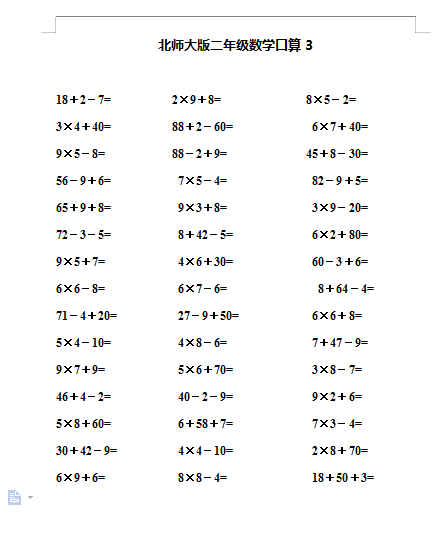18＋2－7=          2×9＋8=              8×5－2=

3×4＋40=          88＋2－60=             6×7＋40=

9×5－8=           88－2＋9=             45＋8－30=

56－9＋6=           7×5－4=              82－9＋5=

65＋9＋8=           9×3＋8=              3×9－20=

72－3－5=           8＋42－5=             6×2＋80=

2022：新北师大版小学数学2年级下册口算 (4)（doc文档1电页子档下载）